# Blackboard Shots with Prefix "Severa"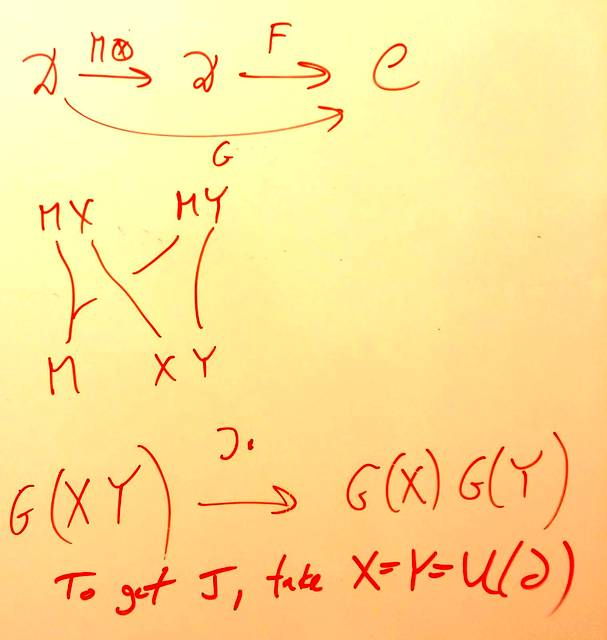131205-104541: Getting the twist.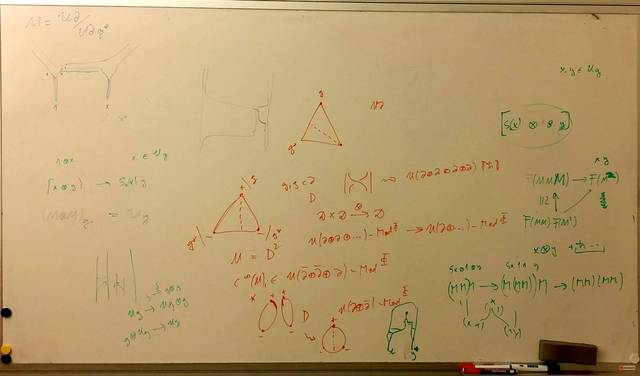131205-100511: Explicit quantization formulas.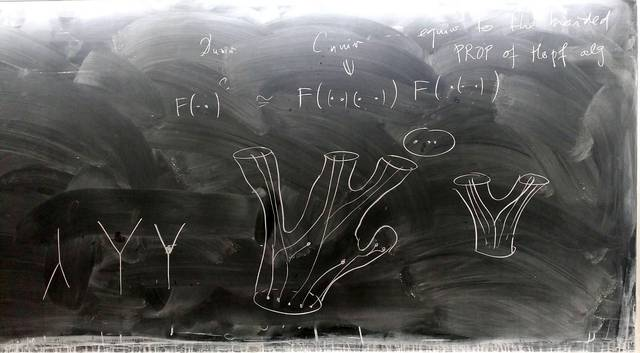131204-045438: Quantizing Lie bialgebras - the universal construction (4).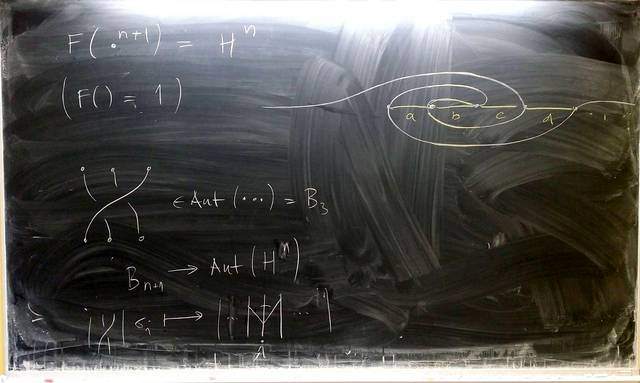131204-045437: Quantizing Lie bialgebras - the universal construction (3).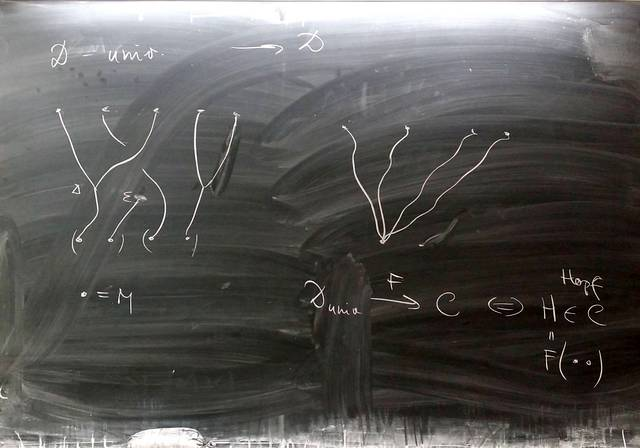131204-045436: Quantizing Lie bialgebras - the universal construction (2).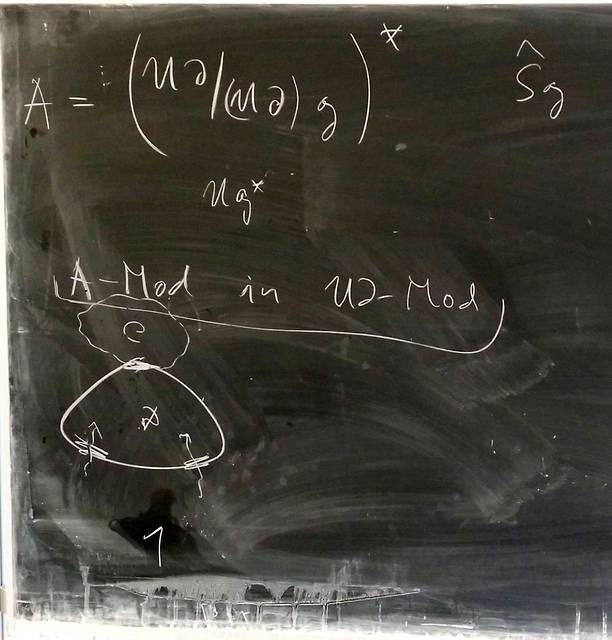131204-045435: Quantizing Lie bialgebras - the universal construction.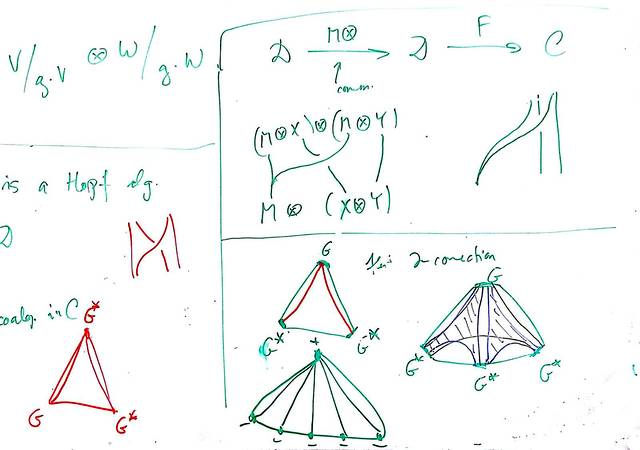131128-024041: Quantizing Lie bialgebras (2).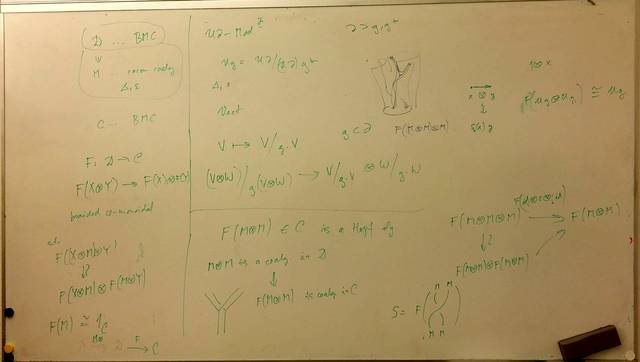131125-065032: Quantizing Lie bialgebras.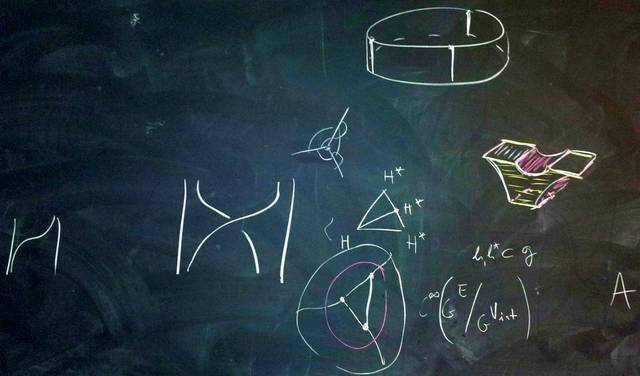131001-080619: Quantizing quasi-Poisson structures (2).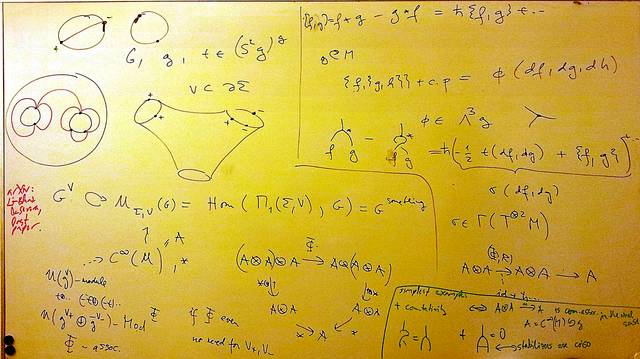130927-105612: Quantizing quasi-Poisson structures.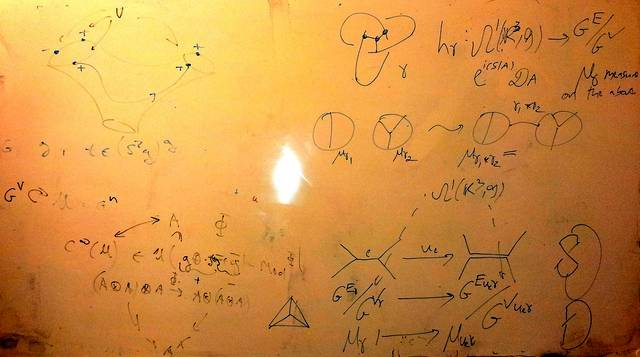130924-105132: Some group level interpretations of associators.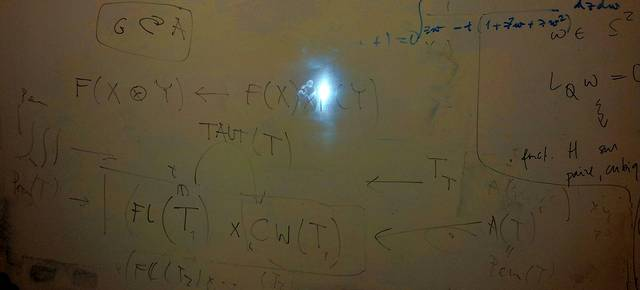130924-102114: A groupoid interpretation of $FL\times CW$.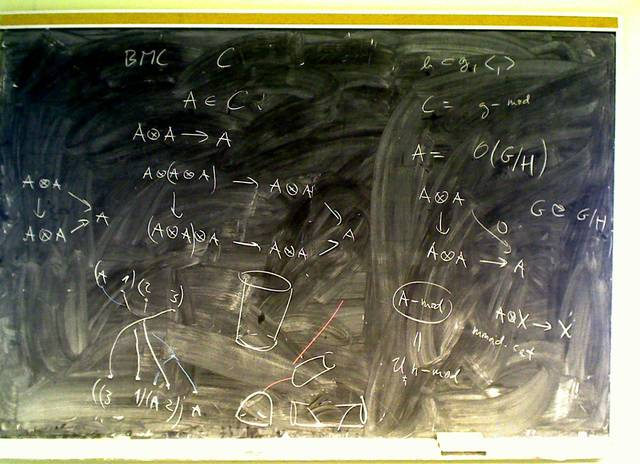110202-112837: The $A$ in Etingof-Kazhdan.110131-184732: The Kontsevich vanishing lemma (3).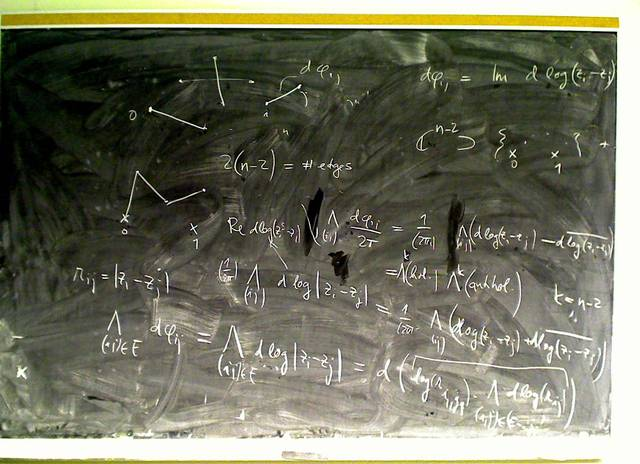110131-174434: The Kontsevich vanishing lemma (2).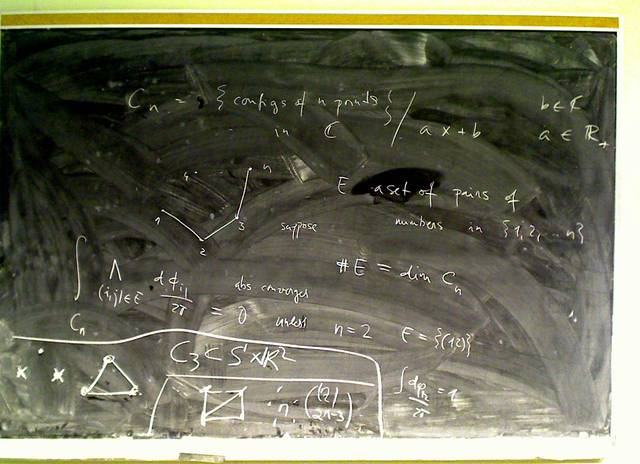110131-172714: The Kontsevich vanishing lemma.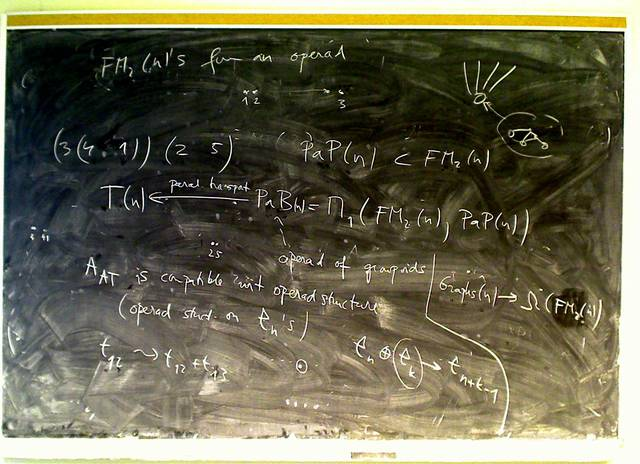110128-110538: $\Phi_{AT}\in{\mathcal A}^{pb}$, day 2 (9).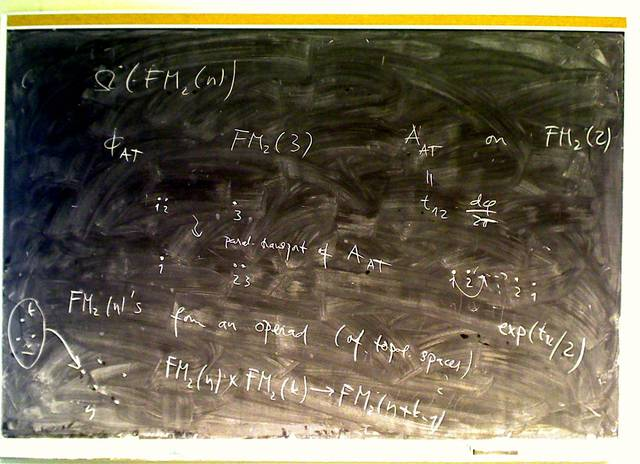110128-104057: $\Phi_{AT}\in{\mathcal A}^{pb}$, day 2 (8).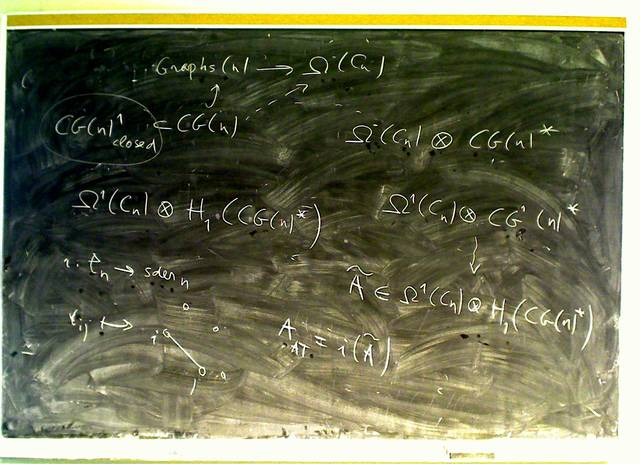110128-103444: $\Phi_{AT}\in{\mathcal A}^{pb}$, day 2 (7).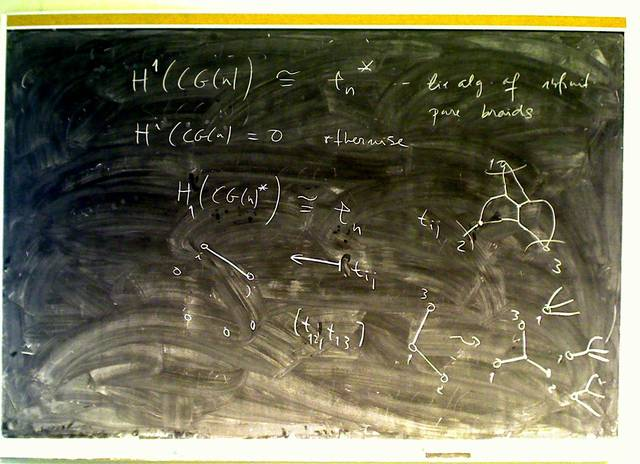110128-102106: $\Phi_{AT}\in{\mathcal A}^{pb}$, day 2 (6). (more...)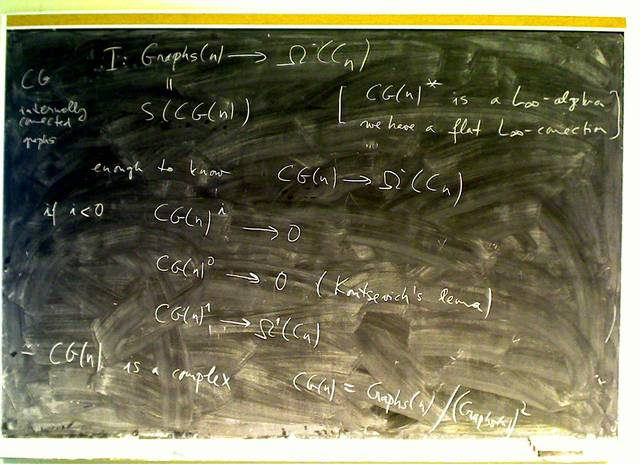110128-100631: $\Phi_{AT}\in{\mathcal A}^{pb}$, day 2 (5).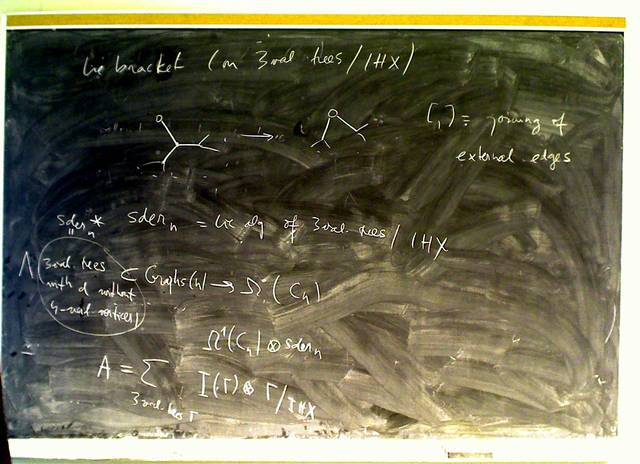110128-095607: $\Phi_{AT}\in{\mathcal A}^{pb}$, day 2 (4).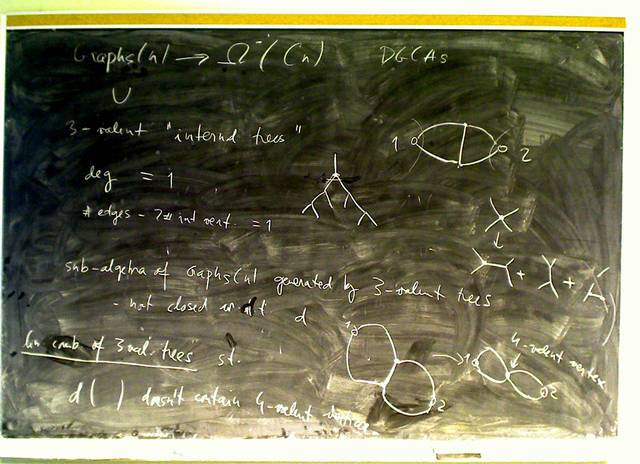110128-094323: $\Phi_{AT}\in{\mathcal A}^{pb}$, day 2 (3).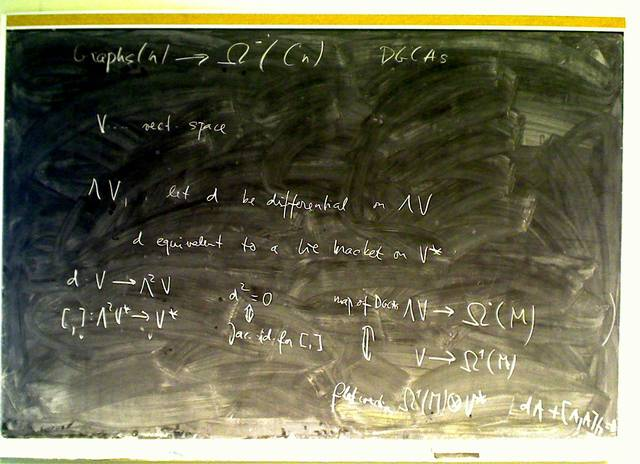110128-093224: $\Phi_{AT}\in{\mathcal A}^{pb}$, day 2 (2).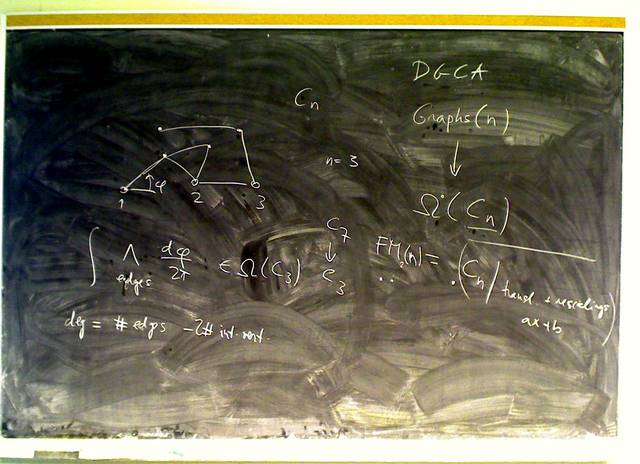110128-092351: $\Phi_{AT}\in{\mathcal A}^{pb}$, day 2.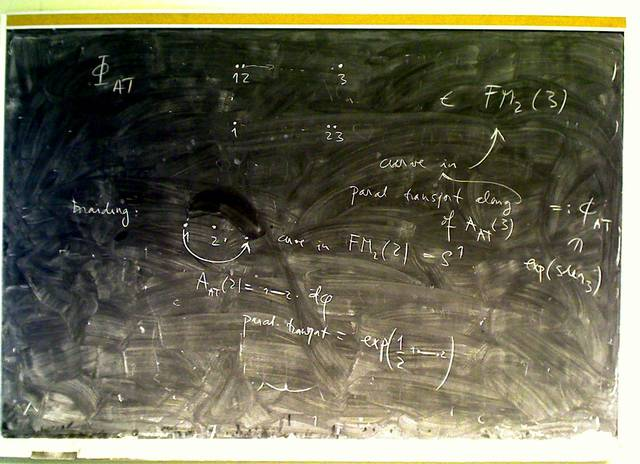110126-110302: $\Phi_{AT}\in{\mathcal A}^{pb}$ (8)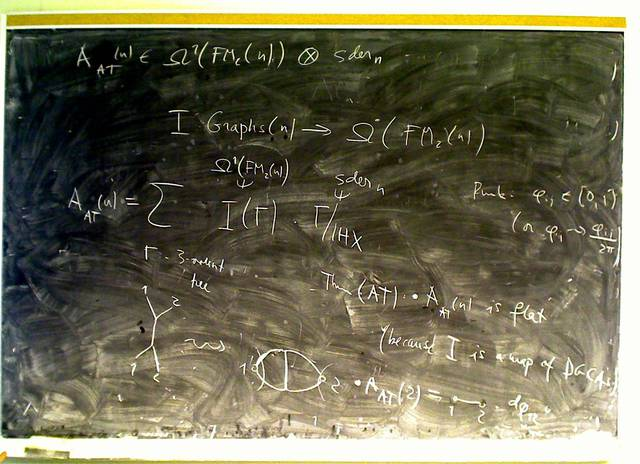110126-105407: $\Phi_{AT}\in{\mathcal A}^{pb}$ (7)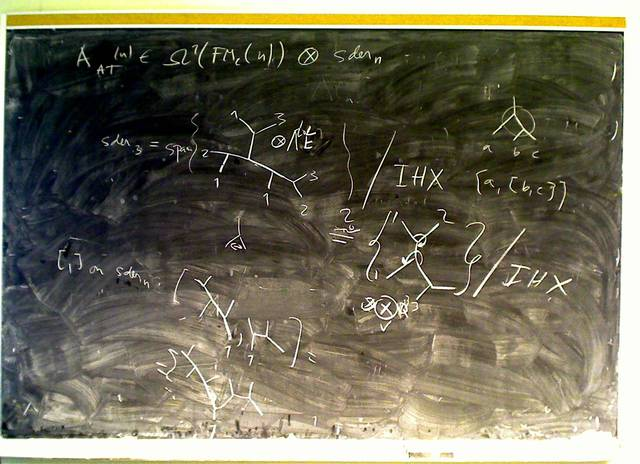110126-104058: $\Phi_{AT}\in{\mathcal A}^{pb}$ (6)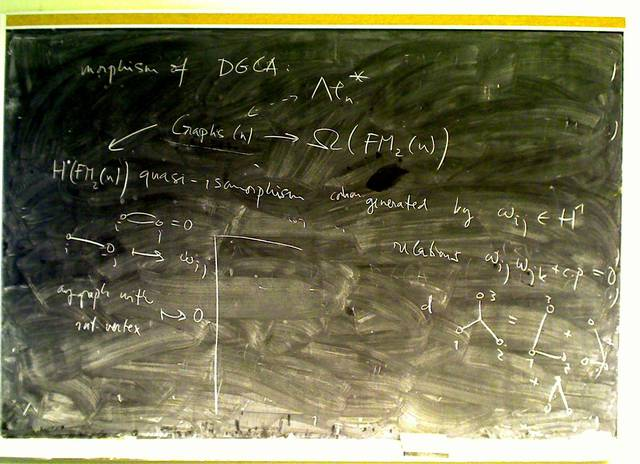110126-102240: $\Phi_{AT}\in{\mathcal A}^{pb}$ (5)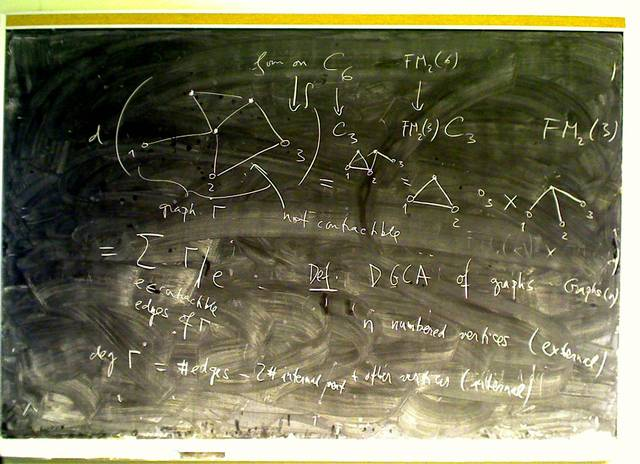110126-100927: $\Phi_{AT}\in{\mathcal A}^{pb}$ (4)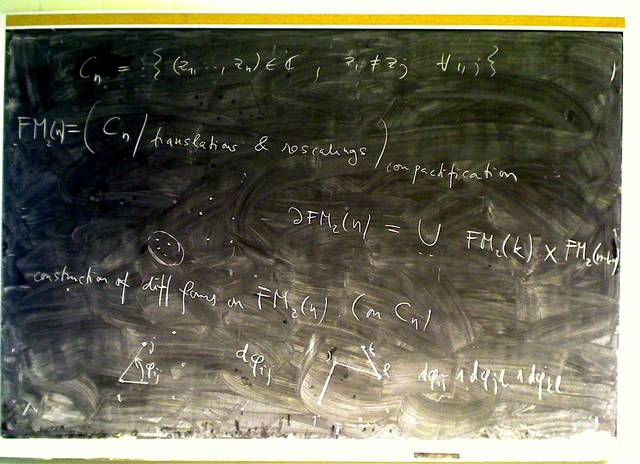110126-095855: $\Phi_{AT}\in{\mathcal A}^{pb}$ (3) (more...)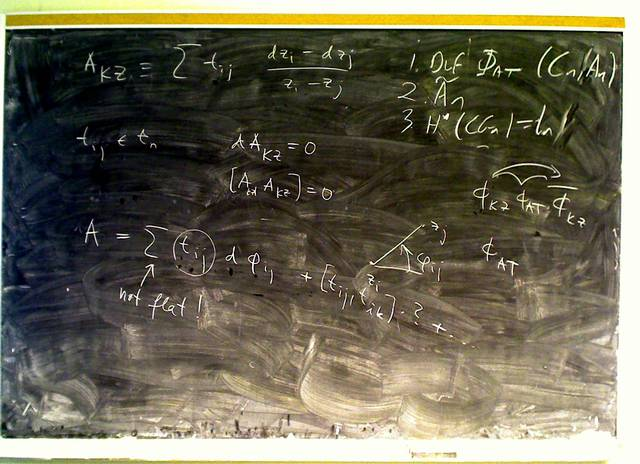110126-095118: $\Phi_{AT}\in{\mathcal A}^{pb}$ (2) (more...)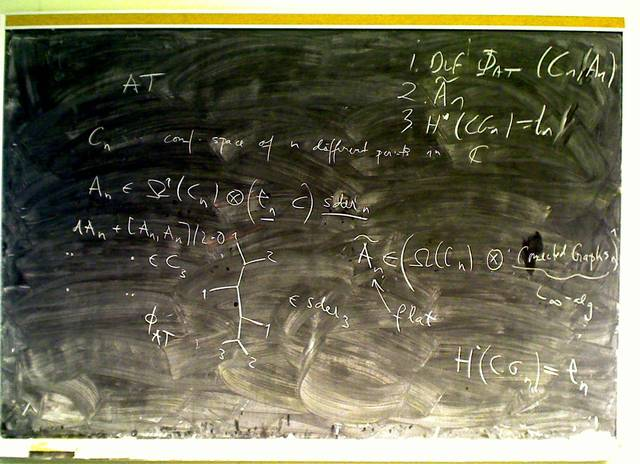110126-093919: $\Phi_{AT}\in{\mathcal A}^{pb}$ (more...)